## Calculate Years Between Dates In Ms Excel

If you are an avid Ms Excel user, then you might have come across a task in which you needed to calculate the years between the dates; you might take it easy and do this task manually, which is also feasible but only for calculating the years between 3 to 4 dates. But when it comes to hundreds of dates, it becomes a cumbersome and time taking task to calculate the years between them manually.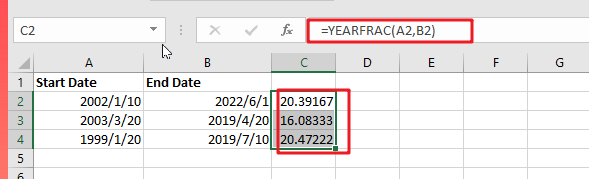But don’t worry since this post will teach you the simplest technique to calculate years between dates in Microsoft Excel. All you have to do is thoroughly read the article.

So without any further ado, let’s dive into it

## General Formula

`=YEARFRAC(start_date,end_date)`

### Explanations Of Syntax:

You must understand the syntax used in this formula in order to apply it to complete your task.

• Parenthesis (): The primary function of this symbol is to group the elements.
• The Comma Symbol (,): This acts as a separator.
• YEARFRAC: It computes the year’s percentage represented by the number of entire days between two dates (start date and end date).

## Summary

The YEARFRAC function, which returns a decimal value indicating the fraction of a year between two dates, may be used to compute the number of years between two dates. The formula in D6 in the example is:

`=YEARFRAC(A2, B2)`

### Explanation

YEARFRAC provides a decimal value that represents the fractional years between two dates. As an example:

`=YEARFRAC("January 1, 2020","January 1, 2022") / yields 2`

Here are a few instances of YEARFRAC’s calculated results:

 Start Date End Date YEARFRAC result 2002/1/10 2022/6/1 20.39167 2003/3/20 2019/4/20 16.08333 1999/1/20 2019/7/10 20.47222

The formula in C2 in the example is: `=YEARFRAC(A2, B2)` / returns 20 Rounding outcome

You can round the number after you obtain the decimal value. For example, you might use the ROUND function to round to the nearest whole number:

`=ROUND(YEARFRAC(A2,B2),0)`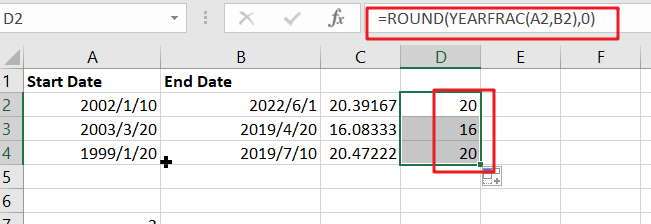Only Whole Years

You may also wish to maintain only the integer component of the result, with no fractional values, to ensure that you only count entire years. In such instance, merely use the INT function to enclose YEARFRAC:

`=INT(YEARFRAC(A2,B2))`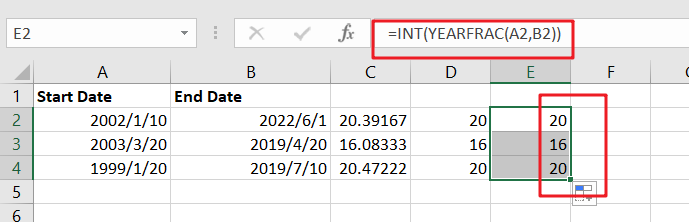Example #2

Assuming you already have the data established in the excel sheets, you do not need to repeat the first step. For those doing it for the very first time, open Excel on your laptop, create a new or empty excel sheet, and record your chosen date values as shown below.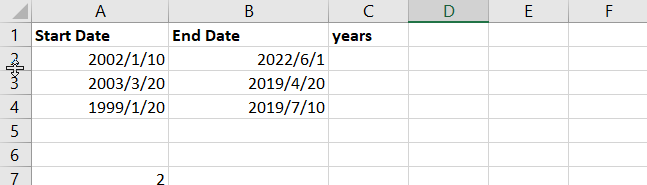We’ll use the YEARFRAC function to calculate the years between the dates on the excel mentioned above. This is the function that computes the number of years between two dates. The YEARFRAC function has the ability to compute in decimal; for example, it can display 1.5 years to imply one and a half years.

Years are where we anticipate recording our outcomes in the column with the title. To compute the years between the dates in cells A2 and B2,

Enter the formula =YEARFRAC (A2, B2) in the formula bar.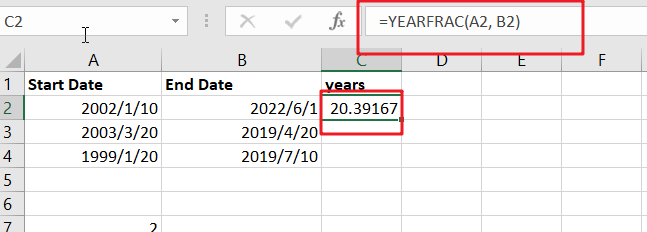Apply the same procedure to the remaining cells, or hold and drag the cursor to compute the remaining years automatically.

Enter this formula in cell D2 and drag the fill handle to get the additional computations.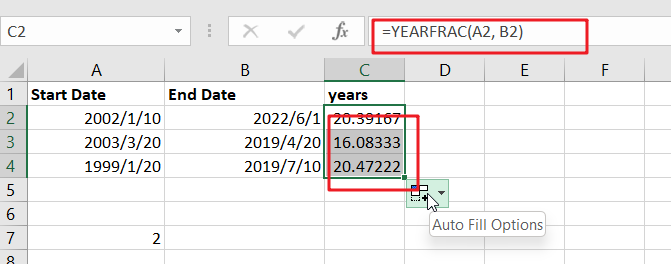To round the decimal or fractional value to the closest whole number, use the ROUND function, such as =ROUND(YEARFRAC(A2, B2),0)the above example shows how to round a fractional value to the nearest whole number.

If we want to return the integer component of the year’s value and not the fractional part, wrap the YEARFRAC function inside the INT function, as in =INT(YEARFRAC(B2, C2)). Figure 4: Obtaining the Year Value Absent Fractional ValueNote: There is an optional third input to the YEARFRAC function that determines how days are tallied when computing fractional years. The default approach is to calculate days between two dates using a 360-day year, with each month having 30 days.

### Related Functions

• Excel INT function
The Excel INT function returns the integer portion of a given number. And it will rounds a given number down to the nearest integer.The syntax of the INT function is as below:= INT (number)…
• Excel Round function
The Excel INT function rounds a number to a specified number of digits. You can use the ROUND function to round to the left or right of the decimal point in Excel.The syntax of the ROUND function is as below:=ROUND (number, num_digits)…

## How to calculate age from date of birth

This post explains that how to get age from the date of birth in a Cell. How to calculate birthdate to age using formulas in Excel. How to convert the birth of date to age quickly in Excel.

## Calculate age from date of birth

If you have a table that contains birthdate data in a range of Cells in Excel, and you want to get the age from those people’s birthdate value, you can use a combination of the INT function, the TODAY function to create an excel formula .

You can use the TODAY function to get the current date and then is subtracted to the value of birthdate and then the number is divided by 365 to get the exact age value, last, using the INT function to convert the age value to the integer.

Assuming that you want to calculate date of birth to age in Cell B2, you can use the following formula:

`=INT((TODAY()-B2)/365)`

Let’s see how this formula works:

=TODAY()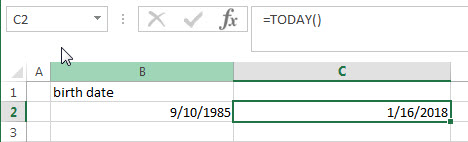This formula returns the current date.

=(TODAY()-B2)/365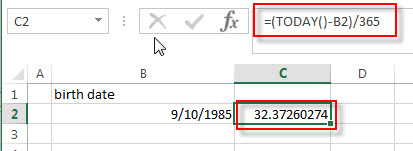The current date subtracts the date of birth to get the number of days of birth.  The returned number is divided by 365 (one year) to get the exact numbers of years.  Then it goes into the INT function as its argument.

=INT((TODAY()-B2)/365)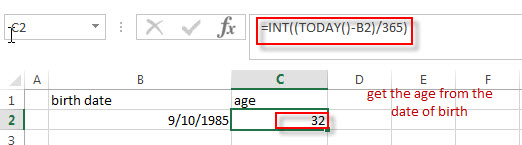This formula will round a decimal down to an integer. So you can get the age from the date of birth.

## Calculate age from date of birth with DATEDIF function

You can also create a formula based on the DATEIF function and TODAY function or NOW function. The DATEDIF function can return the number of complete year between the current date and birth date. So you can write down the following formula to convert date of birth to age quickly:

`=DATEDIF(B2,TODAY(),"Y")`

OR

`=DATEDIF(B2,NOW(),"Y")`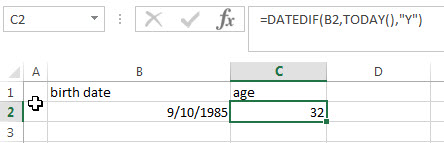## Calculate age from date of birth with YEARFRAC function

You can also create an excel formula based on the INT function or ROUNDDOWN function, the YEARFRAC function and the TODAY function to calculate age from birth of date.

The YEARFRAC function can be used to calculate the fraction of the year represented by the number of whole days between the date of birth and the current date.  Then you need to use the INT function or ROUNDDOWN function to round down the number returned by the YEARFRAC function to the integer value.

So you can write down the following formula:

`=INT(YEARFRAC(B2, TODAY(), 1))`

OR

`=ROUNDDOWN(YEARFRAC(B2, TODAY(), 1),0)`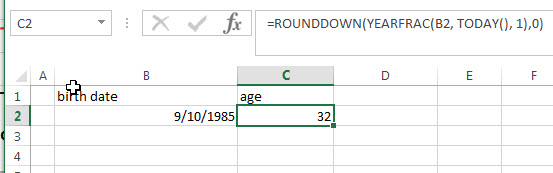### Related Formulas

• Convert Date to Day of Week in Excel
If you want to get the day name of the week as an abbreviation from a date, such as: Mon, Tue, etc. Enter the following TEXT formula in the Cell C1 that you want to get the day name of the week…
• Convet Text Date dd/mm/yy to mm/dd/yyyy as Date Format
If you want to convert dates in the text format to the date format as mm/dd/yyyy, you can use a combination of the DATE function, the VALUE function, the RIGHT function, the MID function, and the LEFT function to create a new excel formula….
• Convert date to month name with Text Function
If you want to convert the date to a month name, you can use the TEXT function with a specified format code like “mmm”in excel.  You can try to run the following TEXT formula:=TEXT(B1,”mmm”)…
• Convert Date to text with Text Function in Excel
you can use TEXT function in excel to convert dates to text in a specific format code. For example, The TEXT function can use the following patterns, like as: “mm/dd/yyyy”, “yyyy/mm/dd”, etc…
• Convert date to month and year only in excel
If you want to convert the date to month and year only, you can use “yyyymm” format code within the TEXT function in excel, so you can write down the below TEXT formula:=TEXT(date,”yyyymm”)…,
• Convert date to month and day only in excel
If you want to convert the date (mm/dd/yyyy) to month and day only, you can use “mm dd” format code within the TEXT function in excel, so you can write down the below TEXT formula: =TEXT(date,”mm dd”)…

### Related Functions

• Excel DATEDIF function
The Excel DATEDIF function returns the number of days, months, or years between tow dates.The syntax of the DATEDIF function is as below:=DATEDIF (start_date,end_date,unit)…
• Excel INT function
The Excel INT function returns the integer portion of a given number. And it will rounds a given number down to the nearest integer.The syntax of the INT function is as below:= INT (number)…